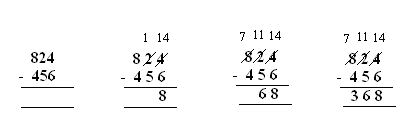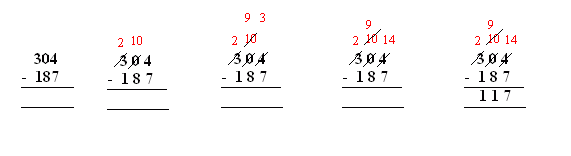Email us to get an instant 20% discount on highly effective K-12 Math & English kwizNET Programs!

#### Online Quiz (WorksheetABCD)

Questions Per Quiz = 2 4 6 8 10

### Grade 4 - Mathematics1.18 Subtraction with Regrouping

 Method: Start subtracting from the ones place. If the subtrahend is lesser than minuend in ones place then we borrow 1 from tens place. If the subtrahend is lesser than minuend in tens place then we borrow 1 from hundreds place Example: Subtraction with regrouping:Start from the ones place, 4 is lesser than 6. So 1 is borrowed from 2 and 4 becomes 14. In tens place, 2 becomes 1, now again 1 is less than 5, so 1 is borrowed from 8 and 1 becomes 11. In ones place 4 is subtracted from 7. Answer: 368 Subtracting across zeros:When there is a zero it is always made 10 by borrrowing 1 from digits in higher place values. In ones place 4 is made 14 and zero is made 9 (since 1 is borrowed from 10 to make 14) In hundreds place 3 becomes 2. Answer: 117 Directions: Subtract the following numbers. Also write at least ten examples of your own.
 Q 1: 2,308 - 924 =Answer: Question 2: This question is available to subscribers only!